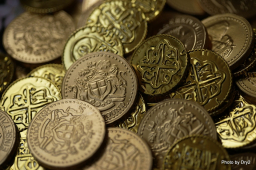# Originally 71464

The father originally wanted to divide the financial amount between his sons in the ratio of 7:6. However, he then split it at 6:5 (in the same order). One of the sons got angry that he should have received 120 euros more. How many euros did each son receive?

a =  9360 Eur
b =  7800 Eur

### Step-by-step explanation:Did you find an error or inaccuracy? Feel free to write us. Thank you!

Tips for related online calculators
Need help calculating sum, simplifying, or multiplying fractions? Try our fraction calculator.
Check out our ratio calculator.
Do you have a linear equation or system of equations and looking for its solution? Or do you have a quadratic equation?